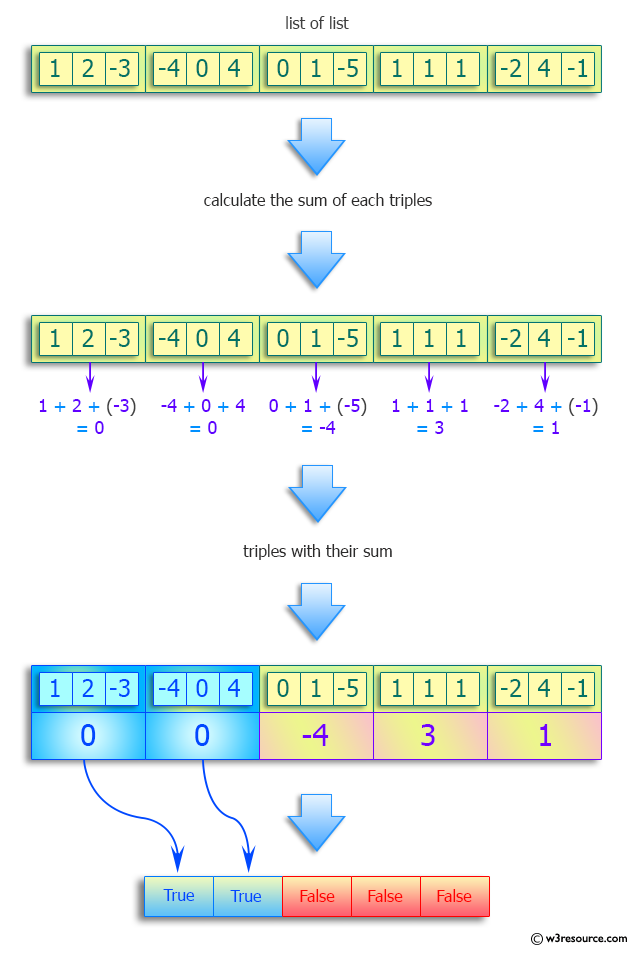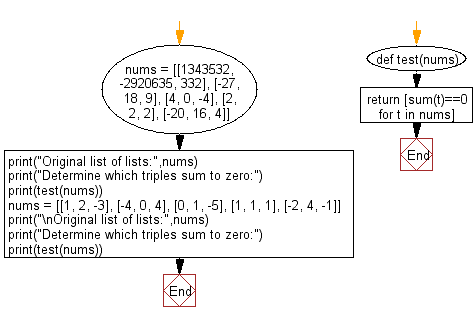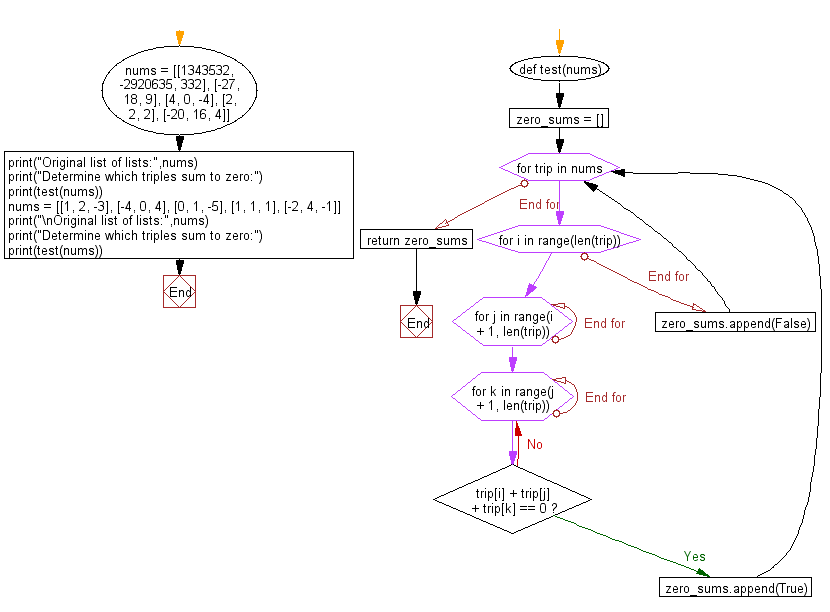﻿ Python: Determine which triples sum to zero - w3resource# Python: Determine which triples sum to zero

## Python Programming Puzzles: Exercise-39 with Solution

Write a Python program to determine which triples sum to zero from a given list of lists.

```Input:
[[1343532, -2920635, 332], [-27, 18, 9], [4, 0, -4], [2, 2, 2], [-20, 16, 4]]
Output:
[False, True, True, False, True]

Input:
[[1, 2, -3], [-4, 0, 4], [0, 1, -5], [1, 1, 1], [-2, 4, -1]]
Output:
[True, True, False, False, False]
```

Pictorial Presentation:Sample Solution-1:

Python Code:

``````#License: https://bit.ly/3oLErEI

def test(nums):
return [sum(t)==0 for t in nums]

nums = [[1343532, -2920635, 332], [-27, 18, 9], [4, 0, -4], [2, 2, 2], [-20, 16, 4]]
print("Original list of lists:",nums)
print("Determine which triples sum to zero:")
print(test(nums))
nums = [[1, 2, -3], [-4, 0, 4], [0, 1, -5], [1, 1, 1], [-2, 4, -1]]
print("\nOriginal list of lists:",nums)
print("Determine which triples sum to zero:")
print(test(nums))
``````

Sample Output:

```Original list of lists: [[1343532, -2920635, 332], [-27, 18, 9], [4, 0, -4], [2, 2, 2], [-20, 16, 4]]
Determine which triples sum to zero:
[False, True, True, False, True]

Original list of lists: [[1, 2, -3], [-4, 0, 4], [0, 1, -5], [1, 1, 1], [-2, 4, -1]]
Determine which triples sum to zero:
[True, True, False, False, False]
```

Flowchart:## Visualize Python code execution:

The following tool visualize what the computer is doing step-by-step as it executes the said program:

Sample Solution-2:

Python Code:

``````#License: https://bit.ly/3oLErEI

def test(nums):
zero_sums = []
for trip in nums:
for i in range(len(trip)):
for j in range(i + 1, len(trip)):
for k in range(j + 1, len(trip)):
if trip[i] + trip[j] + trip[k] == 0:
zero_sums.append(True)
break
else:
continue
break
else:
continue
break
else:
zero_sums.append(False)
return zero_sums

nums = [[1343532, -2920635, 332], [-27, 18, 9], [4, 0, -4], [2, 2, 2], [-20, 16, 4]]
print("Original list of lists:",nums)
print("Determine which triples sum to zero:")
print(test(nums))
nums = [[1, 2, -3], [-4, 0, 4], [0, 1, -5], [1, 1, 1], [-2, 4, -1]]
print("\nOriginal list of lists:",nums)
print("Determine which triples sum to zero:")
print(test(nums))
``````

Sample Output:

```Original list of lists: [[1343532, -2920635, 332], [-27, 18, 9], [4, 0, -4], [2, 2, 2], [-20, 16, 4]]
Determine which triples sum to zero:
[False, True, True, False, True]

Original list of lists: [[1, 2, -3], [-4, 0, 4], [0, 1, -5], [1, 1, 1], [-2, 4, -1]]
Determine which triples sum to zero:
[True, True, False, False, False]
```

Flowchart:## Visualize Python code execution:

The following tool visualize what the computer is doing step-by-step as it executes the said program:

Python Code Editor :

Have another way to solve this solution? Contribute your code (and comments) through Disqus.

What is the difficulty level of this exercise?

Test your Programming skills with w3resource's quiz.

﻿

## Python: Tips of the Day

Decapitalizes the first letter of a string:

Example:

```def tips_decapitalize(s, upper_rest=False):
return s[:1].lower() + (s[1:].upper() if upper_rest else s[1:])
print(tips_decapitalize('PythonTips'))
print(tips_decapitalize('PythonTips', True))
```

Output:

```pythonTips
pYTHONTIPS
```